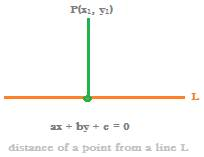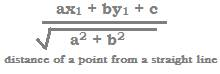## Distance between

Related to ‘Distance between’, we will discuss below three types of formulas as found in the math topic of coordinate geometry.

The three formulas related to ‘Distance between’ in coordinate geometry are as follows:

1. Distance between two points
2.
3. Distance between two straight lines and
4.
5. Distance between a point and a straight line.
6.

1. Distance between two points:

Consider two points A and B in the rectangular   coordinate system formed by the x-axis and the y-axis.

Let the coordinates of the point:

A be (x1, y1) and those of point B be (x2, y2)

The formula for finding distance between two points

A (x1, y1) and B(x2, y2) is

Example:

What is the distance between the two points A (2, 3) and B (4, 5)

Solution:

From the above formula, the distance between the two points

A (2, 3) and B (4, 5) is

2. Distance between two straight lines

Consider two straight lines L and M in the Cartesian coordinate plane, which are parallel to each other.

Let the two straight lines L and M, which are parallel to each other be such as

Equation of line L is: ax + by + c = 0, and

Equation of line M is:ax + by + d = 0.

Then the formula for

Distance between the two parallel lines L and M isIn the equations of the two parallel lines L and M:

ax + by + c = 0 and ax + by + d = 0

difference of constant terms of the two equations is c – d, and

coefficient of x = a, and coefficient of y = b

Now, let us solve a question on distance between two straight lines.

Example:

Find the distance between the two straight lines L and M, which are parallel to each other, such that the equation of

L is 3x + 4y + 5 = 0 and that of M is 3x + 4y + 6 = 0

Solution:

In the equations of the two parallel lines L and M,

Difference of the constant terms = 6 – 5 = 1,

And in both of the equations,

Coefficient of x, i.e. a = 3, and coefficient of y, i.e. b = 4.

So, a2 + b2 = 32 + 42 = 9 + 16 = 25

Therefore, distance between the two straight lines L and M is

1/ (√25) = 1/5 units.

3. Distance between a point and a straight line

Consider a straight line L whose equation is ax + by + c = 0

Let there be a point P with coordinates (x, y).

Now, the distance of a point P(x1, y1) from a straight line L is the length of the line segment drawn perpendicular from the point P to the straight line L.And the formula to find the perpendicular distance between a straight line L and a point P (x1, y1) is